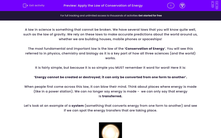# Apply the Law of Conservation of Energy

In this worksheet, students will study the law of the conservation of energy and consider ways of increasing efficiency within systems.Key stage:  KS 4

Year:  GCSE

GCSE Boards:   AQA, AQA Trilogy, AQA Synergy, OCR 21st Century, OCR Gateway, Pearson Edexcel, Eduqas,

Popular topics:   Physics worksheets

Difficulty level:#### Worksheet Overview

A law in science is something that cannot be broken. We have several laws that you will know quite well, such as the law of gravity. We rely on these laws to make accurate predictions about the world around us, whether we are building houses, mobile phones or spaceships!

The most fundamental and important law is the law of the ‘Conservation of Energy’. You will see this referred to in physics, chemistry and biology as it is a key part of how all three sciences (and the world) works.

It is fairly simple, but because it is so simple you MUST remember it word for word! Here it is:

‘Energy cannot be created or destroyed; it can only be converted from one form to another’.

When people first come across this law, it can blow their mind. Think about places where energy is made (like in a power station). We can no longer say energy is made -  we can only say that energy is transferred.

Let’s look at an example of a system (something that converts energy from one form to another) and see if we can spot the energy transfers that are taking place.Look at this light bulb.

What energy is going into it? Electrical.

The energy store that is depleting is the electrical store. This is because electrical energy is being converted into light energy and heat energy. We want the light energy, this is useful, but we also get some wasted energy as heat. We didn’t design a light bulb to get hot, but it does anyway. This is what we call wasted energy as it is not doing the job that we wanted it to do.

When we compare the amount of energy we want with the amount of energy we don’t want, we come up with a number that shows efficiency.  Efficiency is a simple measurement telling us how much of the energy is going into doing the job that we have designed it for. It can be presented as a number between 0 and 1 (where 0 is none of the energy going into what we want, and 1, where all of the energy is going into what we want) or as a percentage.

FUN FACT – it is impossible to get a system that has an efficacy of 100%. Not just difficult, not just that we have never managed to achieve it, but impossible – it is another one of those laws.

Let's look at how you work out efficiency then:So, let’s try an example:

A light bulb takes in 100 J of energy every second. 40 J of that energy are given off as light. Calculate the efficiency of the light bulb.

Step 1  Highlight all of the numbers in the question.

A light bulb takes in 100 J of energy every second. 40 J of that energy are given off as light. Calculate the efficiency of the light bulb.

Step 2  Find out what each value is.

Energy in = 100 J

Useful energy out = 40 J

Step 3  Put the number into the equation.

Efficiency = 40 ÷ 100

Step 4  Press = and get your number (if it asks for a percentage, all you need to do is x 100 at the very end).

Efficiency  = 0.4 or 40%

There are no units for efficiency, but if it is a percentage, always put the percentage sign after it.

Finally, we need to look at how to manage efficiency within a system. This will vary from system to system, but you need to look for where there is energy loss and how you can manage that energy loss. A really common example is energy lost as friction in most systems. Let's say you skateboard, but your board isn't very fast or it stops too quickly. This is due to energy loss in the friction of the wheels and the bearings.  We can try to minimise this loss by oiling the wheels, which will reduce the loss of energy from the heating effect of friction. You will now go further with your nicely oiled wheels - brilliant!

Now it's time for some questions.

### What is EdPlace?

We're your National Curriculum aligned online education content provider helping each child succeed in English, maths and science from year 1 to GCSE. With an EdPlace account you’ll be able to track and measure progress, helping each child achieve their best. We build confidence and attainment by personalising each child’s learning at a level that suits them.

Get started